### Home > CALC > Chapter 8 > Lesson 8.1.2 > Problem8-20

8-20.
1. Evaluate the following limits. Homework Help ✎

1.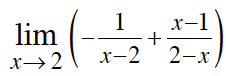2.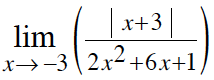3.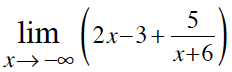4.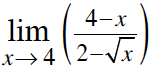5.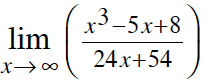6.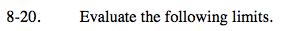$= \lim_{x\rightarrow 2}\left ( -\frac{1}{x-2} \right )+\lim_{x\rightarrow 2}\left ( \frac{x-1}{2-x} \right )$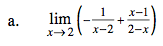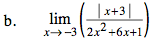Factor the denominator and rewrite the numerator as a piecewise. Notice that the pieces might or might not meet at x = 3.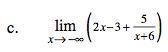What does the graph look like as x→ − ∞?

$\text{What is the end behavior of }y=2x-3+\frac{5}{x+6}?$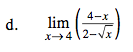Before taking the limit, either factor the numerator multiply the top and bottom by the conjugate of the bottom.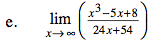Limit → ∞. Compare the highest powers of the top and the bottom. What does this graph look like at the end?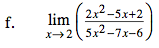Before taking the limit, factor.